### Home > CALC > Chapter 7 > Lesson 7.4.2 > Problem7-190

7-190.
1. 7-190.No Calculator! Evaluate the following limits. Homework Help ✎

1.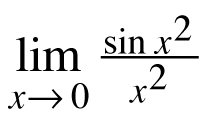2.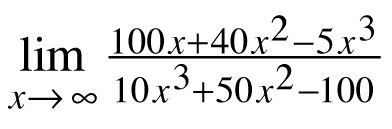3.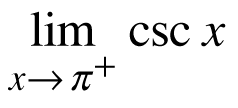4.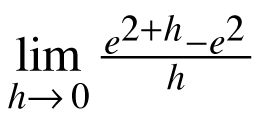5.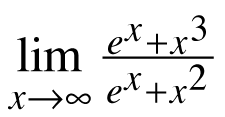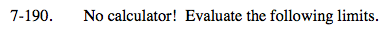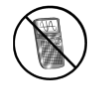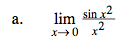$\text{This is an indeterminate form: }\frac{0}{0}.$

Use l'Hopital's Rule.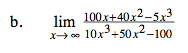This is a limit → ∞.
Compare the powers on the top and bottom only.
Do not neglect their coefficients.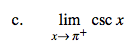$\text{csc}x=\frac{1}{\text{sin}x}\text{ and sin}\pi =0.$

So this limit must be approaching +∞ or −∞.
Which one?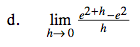This is Hana's Definition of the Derivative.
The limit is equal to f '(2) when f(x) = ex.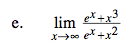This is a limit → ∞. Be careful. Which is more powerful: an exponential function or a polynomial function?